## 4. 3. 1 Boundary Conditions

Boundary conditions typically set the function at the boundary (Dirichlet boundary condition) or its normal derivative (Neumann boundary condition) to a certain value . For differential equations of higher than second-order, e.g. the BiLaplace equation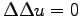, higher order derivatives can be given.

With an identical formulation but with a modified functional meaning, the discretized differential equation can be applied to a boundary vertex or another boundary element. Using finite elements for the Poisson equation, the application of the discretization formula for the interior to a boundary point implicitly yields zero Neumann boundary conditions. This property of finite element methods is called natural boundary condition. The same holds true for the discretization of the Poisson equation using finite volume schemes.

In combination with the boundary conditions some problems occur which can be handled using the method of line-wise assembly rather than the method of element-wise assembly.

For the application of boundary conditions two different methods are available. The first and easiest method is to assign the respective function which is collocated with a boundary point a given value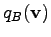. In line-wise assembly, this can be carried out easily by inserting a (trivial) boundary expression or equation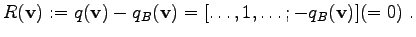(4.38)

In order to reduce the numerical effort, simple boundary conditions such as zero Neumann and Dirichlet conditions are eliminated and directly inserted in the interior equations. In simple cases this can speed up the calculations and reduce the size required for storing matrix coefficients.

For Dirichlet boundary conditions the solution variables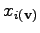for boundary points are replaced by the boundary valueso that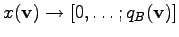. Other boundary conditions can only be eliminated in special cases, for instance, if natural boundary conditions are applied.

Finite differences allow to eliminate all boundary conditions. These conditions are derived using the same scheme as shown in Section 4.3.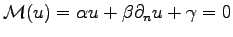(4.39)

A (linearized) expression in the following manner is retrieved, where for each boundary vertex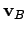exactly one interior vertex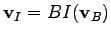can be obtained. The boundary functional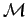is specified by the following equation: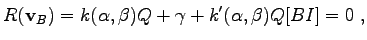(4.40)

where the affine residual expression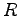for the boundary condition contains the boundary variable as well as unknown variables from the interior of the equation system. For the determination of the normal derivative at the boundary no other boundary vertices are required. One obtains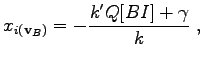(4.41)

The Dirichlet boundary condition and the natural boundary condition are most simple to treat, because either a point can be eliminated or no special treatment has to be performed at all.

In many cases, such as in device simulation, a constant flux or current is required through a certain boundary region. In such a case it is assumed that the connection between the circuit and the device is ideal (i.e. all contacts are at the same potential). In order to obtain the boundary equations for all elements (in general vertices), first all elements at the boundary have the same value - in this case - for the potential. From the set of boundary conditions one element is chosen. This can be accomplished by a topological function that assigns each vertexa vertex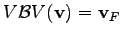. For all boundary verticesbut one first vertex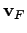the following equation is given: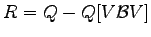(4.42)

For the boundary vertexthe flux boundary condition is given. In finite volumes, this can be specified in the following manner: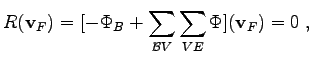(4.43)

whereis the respective boundary region and the function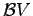determines all vertices incident to the boundary region.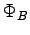denotes the given current which flows through the boundary region.

When assembling this system, the variables corresponding to the boundary vertices can be reduced to one variable which contains the potential of the boundary vertex(and of course the potential of all other points).

Michael 2008-01-16